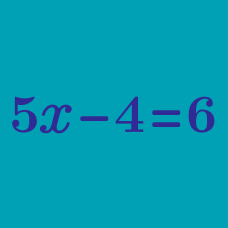Algebra

# Setting Up Equations

On a 2-day roadtrip, Janet drove $210$ miles the first day, and $S$ miles on the second day. She drove a total of $570$ miles.

Which of the following mathematical statements expresses the above?

For his exercise, James runs $D$ miles every weekday, $E$ miles on Saturday, and $E$ miles on Sunday. He runs a total of 27 miles each week.

Which of the following mathematical statements represents the above?

Alex and Bella have 24 sweets in total. Bella has 6 sweets more than Alex.

If $A$ represents Alex's sweets, and $B$ represents Bella's, which of the following statements represents the above relationship?

John is 3 years older than Mary, and Mary is 9 years older than Zack.

Let John's age be denoted by $J$, Mary's age be denoted by $M$ and Zack's age be denoted by $Z.$

Which of the following statements represents their age?

Elle's cellphone was fully charged when she got a call from her friend. She used $x$% of the battery while she was on the phone, and her cellphone is 87% charged now.

Which of the following mathematical statements describes the above?

×